# Post Processing¶

Finite Element Modeling post processing tools.

## Field Outputs¶

### Scalar fields¶

class `abapy.postproc.``FieldOutput`(position='node', data=None, labels=None, dti='I', dtf='f')[source]

Scalar output representing a field evaluated on nodes or elements referenced by their labels. A FieldOutput instance cannot be interpreted with its mesh. On initiation, labels and data will be reordered to have labels sorted.

Parameters: position ('node' or 'element') – location of the field evaluation data (list, array.array, numpy.array containing floats) – value of the field where it is evaluated labels (list, array.array, numpy.array containt ints or None.) – labels of the nodes/elements where the field is evaluated. If None, labels will be [1,2,...,len(data)+1] dti ('I', 'H') – int data type in array.array dtf ('f', 'd') – float data type in array.array
```>>> from abapy.postproc import FieldOutput
>>> data = [-1.,5.,3.]
>>> labels = [1,3,2]
>>> fo = FieldOutput(data=data, labels = labels, position = 'node')
>>> print fo # data is sorted by labels
FieldOutput instance
Position = node
Label Data
1     -1.0
2     3.0
3     5.0
>>> print fo[1:2] # slicing
FieldOutput instance
Position = node
Label Data
1     -1.0
>>> print fo # indexing
FieldOutput instance
Position = node
Label Data
2     3.0
>>> print fo[1,3] # multiple indexing
FieldOutput instance
Position = node
Label Data
1     -1.0
3     5.0
>>> print fo*2 # multiplication
FieldOutput instance
Position = node
Label Data
1     -2.0
2     6.0
3     10.0
>>> fo2 = fo**2  #power
>>> print fo2
FieldOutput instance
Position = node
Label Data
1     1.0
2     9.0
3     25.0
>>> print fo * fo2
FieldOutput instance
Position = node
Label Data
1     -1.0
2     27.0
3     125.0
>>> print fo + fo2
FieldOutput instance
Position = node
Label Data
1     0.0
2     12.0
3     30.0
>>> print abs(fo)
FieldOutput instance
Position = node
Label Data
1     1.0
2     3.0
3     5.0
```

Note

If dti=’H’ is chosen, labels are stored as unsigned 16 bits ints. If more than 65k labels are stored, an OverFlow error will be raised.

#### Add/remove/get data¶

`FieldOutput.``add_data`(label, data)[source]
Adds one point to a FieldOutput instance. Label must not already exist in the current FieldOutput, if not so, nothing will be changed. Data and label will be inserted in self.data, self.labels in order to keep self.labels sorted.
```>>> from abapy.postproc import FieldOutput
>>> data = [5.5, 2.2]
>>> labels = [1,4]
>>> temperature = FieldOutput(labels = labels, data = data, position = 'node')
>>> temperature.add_data(2, 5.)
>>> temperature.data # labels are sorted
array('f', [5.5, 5.0, 2.200000047683716])
>>> temperature.labels # data was sorted like labels
array('I', [1L, 2L, 4L])
```
Parameters: label – labels of the nodes/elements where the field is evaluated. data (float) – value of the field where it is evaluated
`FieldOutput.``get_data`(label)[source]

Returns data at a location with given label.

Parameters: label (int > 0) – location’s label. float

Note

Requesting data at a label that does not exist in the instance will just lead in a warning but if label is negative or is not int, then an Exception will be raised.

#### VTK Export¶

`FieldOutput.``dump2vtk`(name='fieldOutput', header=True)[source]

Converts the FieldOutput instance to VTK format which can be directly read by Mayavi2 or Paraview. This method is very useful to quickly and efficiently plot 3D mesh and fields.

Parameters: name (string) – name used for the field in the output. header (boolean) – if True, adds the location header (eg. CELL_DATA / POINT_DATA) string
```from abapy.postproc import FieldOutput
from abapy.mesh import Mesh, Nodes
x = [0.,1.,0.]
y = [0.,0.,1.]
z = [0.,0.,0.]
labels = [1,2,3]
nodes = Nodes(x=x,y=y,z=z, labels=labels)
mesh = Mesh(nodes=nodes)
mesh.add_element(label = 1 , connectivity = [1,2,3], space = 2 , name = 'tri3') # triangle element
nodeField = FieldOutput()
nodeField.add_data(data = 0., label = 1)
nodeField.add_data(data = 10., label = 2)
nodeField.add_data(data = 20., label = 3)
elementField = FieldOutput(position='element')
elementField.add_data(label = 1, data =10.)
out = ''
out+=mesh.dump2vtk()
out+=nodeField.dump2vtk('nodeField')
out+=elementField.dump2vtk('elementField')
f = open("FieldOutput-dump2vtk.vtk", "w")
f.write(out)
f.close()
```

Result in Paraview:### Vector fields¶

class `abapy.postproc.``VectorFieldOutput`(data1=None, data2=None, data3=None, position='node', dti='I', dtf='f')[source]

3D vector field output. Using this class instead of 3 scalar FieldOutput instances is efficient because labels are stored only since and allows all vector operations like dot, cross, norm.

Parameters: data1 (FieldOutput instance or None) – x coordinate data2 (FieldOutput instance or None) – y coordinate data3 (FieldOutput instance or None) – z coordinate position ('node' or 'element') – position at which data is computed dti ('I' for uint32 or 'H' for uint16) – array.array int data type dtf ('f' float32 or 'd' for float64) – array.array int data type
```>>> from abapy.postproc import FieldOutput, VectorFieldOutput
>>> data1 = [1,2,3,5,6,0]
>>> data2 = [1. for i in data1]
>>> labels = range(1,len(data1)+1)
>>> fo1, fo2 = FieldOutput(labels = labels, data=data1, position='node' ), FieldOutput(labels = labels, data=data2,position='node')
>>> vector = VectorFieldOutput(data1 = fo1, data2 = fo2 )
>>> vector2 = VectorFieldOutput(data2 = fo2 )
>>> vector # short description
<VectorFieldOutput instance: 6 locations>
>>> print vector # long description
VectorFieldOutput instance
Position = node
Label Data1   Data2   Data3
1     1.0     1.0     0.0
2     2.0     1.0     0.0
3     3.0     1.0     0.0
4     5.0     1.0     0.0
5     6.0     1.0     0.0
6     0.0     1.0     0.0
>>> print vector # Returns a VectorFieldOutput instance
VectorFieldOutput instance
Position = node
Label Data1   Data2   Data3
6     0.0     1.0     1.0
>>> print vector[1,4,6] # Picking label by label
VectorFieldOutput instance
Position = node
Label Data1   Data2   Data3
1     1.0     1.0     1.0
4     5.0     1.0     1.0
6     0.0     1.0     1.0
>>> print vector[1:6:2] # Slicing
VectorFieldOutput instance
Position = node
Label Data1   Data2   Data3
1     1.0     1.0     1.0
3     3.0     1.0     1.0
5     6.0     1.0     1.0
>>> vector.get_data(6) # Returns 3 floats
(0.0, 1.0, 0.0)
>>> vector.norm() # Returns norm
<FieldOutput instance: 6 locations>
>>> vector.sum() # returns the sum of coords
<FieldOutput instance: 6 locations>
>>> vector * vector2 # Itemwise product (like numpy, unlike matlab)
<VectorFieldOutput instance: 6 locations>
>>> vector.dot(vector2) # Dot/Scalar product
<FieldOutput instance: 6 locations>
>>> vector.cross(vector2) # Cross/Vector product
<VectorFieldOutput instance: 6 locations>
>>> vector + 2 # Itemwise addition
<VectorFieldOutput instance: 6 locations>
>>> vector * 2 # Itemwise multiplication
<VectorFieldOutput instance: 6 locations>
>>> vector / 2 # Itemwise division
<VectorFieldOutput instance: 6 locations>
>>> vector / vector2 # Itemwise division between vectors (numpy way)
Warning: divide by zero encountered in divide
Warning: invalid value encountered in divide
<VectorFieldOutput instance: 6 locations>
>>> abs(vector) # Absolute value
<VectorFieldOutput instance: 6 locations>
>>> vector ** 2 # Power
<VectorFieldOutput instance: 6 locations>
>>> vector ** vector # Itemwize power
<VectorFieldOutput instance: 6 locations>
```

Note

• data1, data2 and data3 must have same position and label or be None. If one data is None, it is supposed to be zero.
• Storage data dype is the highest standard of all 3 data.
• Numpy is not used in the constructor to allow the creation of instances in Abaqus Python but most other operation require numpy for speed reasons.

#### Add/remove/get data¶

`VectorFieldOutput.``add_data`(label, data1=0.0, data2=0.0, data3=0.0)[source]

Adds one point to a VectorFieldOutput instance. Label must not already exist in the current FieldOutput, if not so, nothing will be changed. Data and label will be inserted in self.data, self.labels in order to keep self.labels sorted.

Parameters: label – labels of the nodes/elements where the field is evaluated. data1 (float) – value of the coordinate 1 of the field where it is evaluated. data2 (float) – value of the coordinate 2 of the field where it is evaluated. data3 (float) – value of the coordinate 3 of the field where it is evaluated.
`VectorFieldOutput.``get_data`(label)[source]

Returns coordinates at a location with given label.

Parameters: label (int > 0) – location’s label. float, float, float

Note

Requesting data at a label that does not exist in the instance will just lead in a warning but if label is negative or is not int, then an Exception will be raised.

`VectorFieldOutput.``get_coord`(number)[source]

Returns a coordinate of the vector as a FieldOutput.

Parameters: number (1,2 or 3) – requested coordinate number, 1 is x and so on. FieldOutput instance
```>>> v1 = Vec.get_coord(1)
```

#### VTK Export¶

`VectorFieldOutput.``dump2vtk`(name='vectorFieldOutput', header=True)[source]

Converts the VectorFieldOutput instance to VTK format which can be directly read by Mayavi2 or Paraview. This method is very useful to quickly and efficiently plot 3D mesh and fields.

Parameters: name (string) – name used for the field in the output. string
```>>> from abapy.postproc import FieldOutput, VectorFieldOutput
>>> from abapy.mesh import RegularQuadMesh
>>> mesh = RegularQuadMesh()
>>> data1 = [2,2,5,10]
>>> data2 = [1. for i in data1]
>>> labels = range(1,len(data1)+1)
>>> fo1, fo2 = FieldOutput(labels = labels, data=data1, position='node' ), FieldOutput(labels = labels, data=data2,position='node')
>>> vector = VectorFieldOutput(data1 = fo1, data2 = fo2 )
>>> out = mesh.dump2vtk() + vector.dump2vtk()
>>> f = open('vector.vtk','w')
>>> f.write(out)
>>> f.close()
```

#### Operations¶

`VectorFieldOutput.``norm`()[source]

Computes norm of the vector at each location and returns it as a scalar FieldOutput.

```>>> norm = Vec.norm()
```
Return type: FieldOutput instance
`VectorFieldOutput.``sum`()[source]

Returns the sum of all coordinates.

Return type: FieldOutput instance
`VectorFieldOutput.``dot`(other)[source]

Returns the dot (i. e. scalar) product of two vector field outputs.

Parameters: other (`VectorFieldOutput`) – Another vector field `FieldOutput`
`VectorFieldOutput.``cross`(other)[source]

Returns the cross product of two vector field outputs.

Parameters: other (`VectorFieldOutput`) – Another vector field `VectorFieldOutput`

### Tensor fields¶

class `abapy.postproc.``TensorFieldOutput`(data11=None, data22=None, data33=None, data12=None, data13=None, data23=None, position='node', dti='I', dtf='f')[source]

Symmetric tensor field output. Using this class instead of 6 scalar FieldOutput instances is efficient because labels are stored only since and allows all operations like invariants, product, sum...

Parameters: data11 (FieldOutput instance or None) – 11 component data22 (FieldOutput instance or None) – 22 component data33 (FieldOutput instance or None) – 33 component data12 (FieldOutput instance or None) – 12 component data13 (FieldOutput instance or None) – 13 component data23 (FieldOutput instance or None) – 23 component position ('node' or 'element') – position at which data is computed dti ('I' for uint32 or 'H' for uint16) – array.array int data type dtf ('f' float32 or 'd' for float64) – array.array int data type
```>>> from abapy.postproc import FieldOutput, TensorFieldOutput, VectorFieldOutput
>>> data11 = [1., 1., 1.]
>>> data22 = [2., 4., -1]
>>> data12 = [1., 2., 0.]
>>> labels = range(1,len(data11)+1)
>>> fo11 = FieldOutput(labels = labels, data=data11,position='node')
>>> fo22 = FieldOutput(labels = labels, data=data22,position='node')
>>> fo12 = FieldOutput(labels = labels, data=data12,position='node')
>>> tensor = TensorFieldOutput(data11 = fo11, data22 = fo22, data12 = fo12 )
>>> tensor2 = TensorFieldOutput(data11= fo22 )
>>> tensor
<TensorFieldOutput instance: 3 locations>
>>> print tensor
TensorFieldOutput instance
Position = node
Label Data11  Data22  Data33  Data12  Data13  Data23
1     1.0e+00 2.0e+00 0.0e+00 1.0e+00 0.0e+00 0.0e+00
2     1.0e+00 4.0e+00 0.0e+00 2.0e+00 0.0e+00 0.0e+00
3     1.0e+00 -1.0e+00        0.0e+00 0.0e+00 0.0e+00 0.0e+00
>>> print tensor[1,2]
TensorFieldOutput instance
Position = node
Label Data11  Data22  Data33  Data12  Data13  Data23
1     1.0e+00 2.0e+00 0.0e+00 1.0e+00 0.0e+00 0.0e+00
2     1.0e+00 4.0e+00 0.0e+00 2.0e+00 0.0e+00 0.0e+00
>>> print tensor *2. + 1.
TensorFieldOutput instance
Position = node
Label Data11  Data22  Data33  Data12  Data13  Data23
1     3.0e+00 5.0e+00 1.0e+00 3.0e+00 1.0e+00 1.0e+00
2     3.0e+00 9.0e+00 1.0e+00 5.0e+00 1.0e+00 1.0e+00
3     3.0e+00 -1.0e+00        1.0e+00 1.0e+00 1.0e+00 1.0e+00
>>> print tensor ** 2 # Piecewise power
TensorFieldOutput instance
Position = node
Label Data11  Data22  Data33  Data12  Data13  Data23
1     1.0e+00 4.0e+00 0.0e+00 1.0e+00 0.0e+00 0.0e+00
2     1.0e+00 1.6e+01 0.0e+00 4.0e+00 0.0e+00 0.0e+00
3     1.0e+00 1.0e+00 0.0e+00 0.0e+00 0.0e+00 0.0e+00
>>> vector = VectorFieldOutput(data1 = fo11)
>>> print tensor * vector # Matrix product
VectorFieldOutput instance
Position = node
Label Data1   Data2   Data3
1     1.0     1.0     0.0
2     1.0     2.0     0.0
3     1.0     0.0     0.0
>>> print tensor * tensor2 # Contracted tensor product
FieldOutput instance
Position = node
Label Data
1     2.0
2     4.0
3     -1.0
```

#### Add/remove/get data¶

`TensorFieldOutput.``add_data`(label, data11=0.0, data22=0.0, data33=0.0, data12=0.0, data13=0.0, data23=0.0)[source]

Adds one point to a VectorFieldOutput instance. Label must not already exist in the current FieldOutput, if not so, nothing will be changed. Data and label will be inserted in self.data, self.labels in order to keep self.labels sorted.

Parameters: label – labels of the nodes/elements where the field is evaluated. data11 – value of the component 11 of the field where it is evaluated. data22 – value of the component 22 of the field where it is evaluated. data33 – value of the component 33 of the field where it is evaluated. data12 – value of the component 12 of the field where it is evaluated. data13 – value of the component 13 of the field where it is evaluated. data23 – value of the component 23 of the field where it is evaluated.
`TensorFieldOutput.``get_data`(label)[source]

Returns the components (11, 22, 33, 12, 13 or 23) at a location with given label.

Parameters: label (int > 0) – location’s label. float, float, float, float, float, float

Note

Requesting data at a label that does not exist in the instance will just lead in a warning but if label is negative or is not int, then an Exception will be raised.

`TensorFieldOutput.``get_component`(number)[source]

Returns a component of the vector as a FieldOutput.

Parameters: number (11, 22, 33, 12, 13 or 23) – requested coordinate number, 1 is x and so on. FieldOutput instance
```>>> v1 = Vec.get_coord(1)
```

#### VTK Export¶

`TensorFieldOutput.``dump2vtk`(name='tensorFieldOutput', header=True)[source]

Converts the TensorFieldOutput instance to VTK format which can be directly read by Mayavi2 or Paraview. This method is very useful to quickly and efficiently plot 3D mesh and fields.

Parameters: name (string) – name used for the field in the output. string

#### Operations and invariants¶

`TensorFieldOutput.``sum`()[source]

Returns the sum of all components of the tensor.

Return type: `FieldOutput` instance.
`TensorFieldOutput.``trace`()[source]

Returns the trace of the tensor: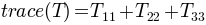Return type: `FieldOutput` instance.
`TensorFieldOutput.``deviatoric`()[source]

Returns the deviatoric part tensor: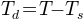Return type: `TensorFieldOutput` instance
`TensorFieldOutput.``spheric`()[source]

Returns the spheric part of the tensor: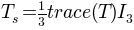Return type: `TensorFieldOutput` instance
`TensorFieldOutput.``i1`()[source]

Returns the first invariant, is equivalent to trace.

Return type: `FieldOutput` instance.
`TensorFieldOutput.``i2`()[source]

Returns the second invariant of the tensor defined as: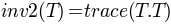Return type: `FieldOutput` instance.

Note

this definition is the most practical one for mechanical engineering but not the only one possible.

`TensorFieldOutput.``i3`()[source]

Returns the third invariant of the tensor: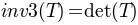Return type: `FieldOutput` instance.
`TensorFieldOutput.``j2`()[source]

Returns the second invariant of the deviatoric part of the tensor defined as: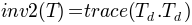Return type: `FieldOutput` instance.

Note

this definition is not the mathematical definition but is the most practical one for mechanical engineering. This should be debated.

`TensorFieldOutput.``j3`()[source]

Returns the third invariant of the deviatoric part of the tensor: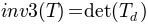Return type: `FieldOutput` instance.
`TensorFieldOutput.``eigen`()[source]

Returns the three eigenvalues with decreasing sorting and the 3 normed respective eigenvectors.

Return type: 3 `FieldOutput` instances and 3 `VectorFieldOutput` instances.
```>>> from abapy.postproc import FieldOutput, TensorFieldOutput, VectorFieldOutput, Identity_like
>>> data11 = [0., 0., 1.]
>>> data22 = [0., 0., -1]
>>> data12 = [1., 2., 0.]
>>> labels = range(1,len(data11)+1)
>>> fo11 = FieldOutput(labels = labels, data=data11,position='node')
>>> fo22 = FieldOutput(labels = labels, data=data22,position='node')
>>> fo12 = FieldOutput(labels = labels, data=data12,position='node')
>>> tensor = TensorFieldOutput(data11 = fo11, data22 = fo22, data12 = fo12 )
>>> t1, t2, t3, v1, v2, v3 = tensor.eigen()
>>> print t1
FieldOutput instance
Position = node
Label       Data
1   1.0
2   2.0
3   1.0
>>> print v1
VectorFieldOutput instance
Position = node
Label       Data1   Data2   Data3
1   0.707106769085  0.707106769085  0.0
2   0.707106769085  0.707106769085  0.0
3   1.0     0.0     0.0
```
`TensorFieldOutput.``pressure`()[source]

Returns the pressure.

Return type: `FieldOutput` instance.
`TensorFieldOutput.``vonmises`()[source]

Returns the von Mises equivalent equivalent stress of the tensor: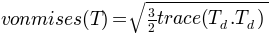Return type: `FieldOutput` instance.
`TensorFieldOutput.``tresca`()[source]

Returns the tresca equivalent stress of the tensor: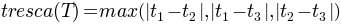where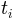is the i-est eigen value of T.

Return type: `FieldOutput` instance.

### Getting field outputs from an Abaqus ODB¶

#### Scalar fields¶

`abapy.postproc.``GetFieldOutput`(odb, step, frame, instance, position, field, subField=None, labels=None, dti='I')[source]

Retrieves a field output in an Abaqus odb object and stores it in a FieldOutput class instance. Field output that are classically available at integration points must be interpolated at nodes. This can be requested in the Abaqus inp file using: Element Output, position = nodes.

Parameters: odb (odb object.) – odb object produced by odbAccess.openOdb in abaqus python or abaqus viewer -noGUI step (string) – step name defined in the abaqus inp file. May be the upper case version of original string name. frame (int) – requested frame indice in the odb. instance (string) – instance name defined in the abaqus odb file. May be the upper case version of the original name. position ('node', 'element') – position at which the output is to be computed. field (string) – requested field output (‘LE’,’S’,’U’,’AC YIELD’,...). subField (string or None) – requested subfield in the case of non scalar fields, can be a component (U1, S12) or an invariant (mises, tresca, inv3, maxPrincipal). In the case of scalar fields, it has to be None labels (list, array.array, numpy.array of unsigned non zero ints or string) – if not None, it provides a set of locations (elements/nodes labels or node/element set label) where the field is to be computed. if None, every available location is used and labels are sorted dti ('I', 'H') – int data type in `array.array` `FieldOutput` instance

Note

This function can only be executed in abaqus python or abaqus viewer -noGUI

```>>> from abapy.postproc import GetFieldOutput
>>> from odbAccess import openOdb
>>> odb = openOdb('indentation.odb')
>>> U2 = GetFieldOutput(odb, step = 'LOADING0', frame = -1, instance ='I_SAMPLE', position =  'node', field = 'U', subField = 'U1') # Gets U2 at all nodes of instance 'I_SAMPLE'
>>> U1 = GetFieldOutput(odb, step = 'LOADING0', frame = -1, instance ='I_SAMPLE', position =  'node', field = 'U', subField = 'U1', labels = [5,6]) # Here labels refer to nodes 5 and 6
>>> S11 = GetFieldOutput(odb, step = 'LOADING0', frame = -1, instance ='I_SAMPLE', position =  'node', field = 'S', subField = 'S11', labels = 'CORE') # Here labels refers to nodes belonging to the node set 'CORE'
>>> S12 = GetFieldOutput(odb, step = 'LOADING0', frame = -1, instance ='I_SAMPLE', position =  'element', field = 'S', subField = 'S12', labels = 'CORE') # Here labels refers to nodes belonging to the node set 'CORE'
```

Note

• If dti=’H’ is chosen, labels are stored as unsigned 16 bits ints. If more than 65k labels are stored, an OverFlow error will be raised.
• This function had memory usage problems in its early version, these have been solved by using more widely array.array. It is still a bit slow but with the lack of numpy in Abaqus, no better solutions have been found yet. I’m open to any faster solution even involving the used temporary rpt files procuced by Abaqus
`abapy.postproc.``MakeFieldOutputReport`(odb, instance, step, frame, report_name, original_position, new_position, field, sub_field=None, sub_field_prefix=None, sub_set_type=None, sub_set=None)[source]

Writes a field output report using Abaqus. The major interrest of this function is that it is really fast compared to `GetFieldOutput` which tends to get badly slow on odbs containing more than 1500 elements. One other interrest is that it doesn’t require to used `position = nodes` option in the INP file to evaluate fields at nodes. It is especially efficient when averaging is necessary (example: computing stress at nodes). The two drawbacks are that it requires `abaqus viewer` (or `cae`) using the `-noGUI` where GetFieldOutput only requires `abaqus python` so it depends on the license server lag (which can be of several seconds). The second drawback is that it requires to write a file in place where you have write permission. This function is made to used in conjunction with `ReadFieldOutputReport`.

Parameters: odb (odb instance produced by `odbAccess.openOdb`) – output database to be used. instance (string) – instance to use. step (string or int) – step to use, this argument can be either the step number or the step label. frame (int) – frame number, can be negative for reverse counting. report_name (string) – name or path+name of the report to written. original_position – position at which the field is expressed. Can be ‘NODAL’, ‘WHOLE_ELEMENT’ or ‘INTEGRATION_POINT’. new_position (string) – position at which you would like the field to be expressed. Can be ‘NODAL’, ‘WHOLE_ELEMENT’ or ‘INTEGRATION_POINT’ or ‘ELEMENT_NODAL’. Note that `ReadFieldOutputReport` will be capable of averaging values over elements when ‘INTEGRATION_POINT’ or ‘ELEMENT_NODAL’ option is sellected. field (string) – field to export, example: ‘S’, ‘U’, ‘EVOL’,... sub_field (string or int) – can be a component of an invariant, example: 11, 2, ‘Mises’, ‘Magnitude’. Here the use of ‘Mises’ instead of ‘MISES’ can be surprising that’s the way abaqus is written.. sub_set (string) – set to which the report is restricted, must be the label of an existing node or element set. sub_set_type (string) – type of the sub_set, can be node or element.

All examples below are performed on a small indentation ODB:

```>>> from odbAccess import openOdb
>>> from abapy.postproc import MakeFieldOutputReport
>>> # Some settings
>>> odb_name = 'indentation.odb'
>>> report_name = 'indentation_core_step0_frame1_S11_nodes.rpt'
>>> step = 0
>>> frame = -1
>>> new_position = 'NODAL'
>>> original_position = 'INTEGRATION_POINT'
>>> field = 'S'
>>> sub_field = 11
>>> instance = 'I_SAMPLE'
>>> sub_set = 'CORE'
>>> sub_set_type = 'element'
>>> # Function testing
>>> odb = openOdb(odb_name)
>>> MakeFieldOutputReport(
...   odb = odb,
...   instance = instance,
...   step = step,
...   frame = frame,
...   report_name = report_name,
...   original_position = original_position,
...   new_position = new_position,
...   field = field,
...   sub_field = sub_field,
...   sub_set_type = sub_set_type,
...   sub_set = sub_set)
>>> new_position = 'INTEGRATION_POINT'
>>> report_name = 'indentation_core_step0_frame1_S11_elements.rpt'
>>> MakeFieldOutputReport(
...   odb = odb,
...   instance = instance,
...   step = step,
...   frame = frame,
...   report_name = report_name,
...   original_position = original_position,
...   new_position = new_position,
...   field = field,
...   sub_field = sub_field,
...   sub_set_type = sub_set_type,
...   sub_set = sub_set)
>>> new_position = 'ELEMENT_NODAL'
>>> report_name = 'indentation_core_step0_frame1_S11_element-nodal.rpt'
>>> MakeFieldOutputReport(
...   odb = odb,
...   instance = instance,
...   step = step,
...   frame = frame,
...   report_name = report_name,
...   original_position = original_position,
...   new_position = new_position,
...   field = field,
...   sub_field = sub_field,
...   sub_set_type = sub_set_type,
...   sub_set = sub_set)
>>> field = 'U'
>>> sub_field = 'Magnitude'
>>> original_position = 'NODAL'
>>> new_position = 'NODAL'
>>> report_name = 'indentation_core_step0_frame1_U-MAG_nodal.rpt'
>>> MakeFieldOutputReport(
...   odb = odb,
...   instance = instance,
...   step = step,
...   frame = frame,
...   report_name = report_name,
...   original_position = original_position,
...   new_position = new_position,
...   field = field,
...   sub_field = sub_field,
...   sub_set_type = sub_set_type,
...   sub_set = sub_set)
```
Four reports were produced:
`abapy.postproc.``ReadFieldOutputReport`(report_name, position='node', dti='I', dtf='f')[source]

Reads a report file generated by Abaqus (for example using `MakeFieldOutputReport` and converts it in FieldOutputFormat.

Parameters: report_name (string) – report_name or path + name of the report to read. position ('node' or 'element') – position where the `FieldOutput` is to be declared. The function will look at the first and the last column of the report. The first will be considered as the label (i. e. element or node) and the last the value. In some case, like reports written using ‘ELEMENT_NODAL’ or ‘INTEGRATION_POINT’ locations, each label will appear several times. The present function will collect all the corresponding values and average them. At the end, the only possibilities for this parameter should be ‘node’ or ‘element’ as described in the doc of `FieldOutput`. dti ('I', 'H') – int data type in array.array dtf ('f', 'd') – float data type in array.array `FieldOutput` instance.

Note

This function can be run either in abaqus python, abaqus viewer -noGUI, abaqus cae -noGUI and regular python.

```>>> from abapy.postproc import ReadFieldOutputReport
>>> report_name = 'indentation_core_step0_frame1_S11_nodes.rpt'
>>> S11 = ReadFieldOutputReport(report_name, position = 'nodes', dti = 'I', dtf = 'f')
```
`abapy.postproc.``GetFieldOutput_byRpt`(odb, instance, step, frame, original_position, new_position, position, field, sub_field=None, sub_field_prefix=None, sub_set_type=None, sub_set=None, report_name='dummy.rpt', dti='I', dtf='f', delete_report=True)[source]

Wraps `MakeFieldOutputReport` and `ReadFieldOutputReport` in a single function to mimic the behavior `GetFieldOutput`.

Parameters: odb (odb instance produced by `odbAccess.openOdb`) – output database to be used. instance (string) – instance to use. step (string or int) – step to use, this argument can be either the step number or the step label. frame (int) – frame number, can be negative for reverse counting. original_position – position at which the field is expressed. Can be ‘NODAL’, ‘WHOLE_ELEMENT’ or ‘INTEGRATION_POINT’. new_position (string) – position at which you would like the field to be expressed. Can be ‘NODAL’, ‘WHOLE_ELEMENT’ or ‘INTEGRATION_POINT’ or ‘ELEMENT_NODAL’. Note that `ReadFieldOutputReport` will be capable of averaging values over elements when ‘INTEGRATION_POINT’ or ‘ELEMENT_NODAL’ option is sellected. position ('node' or 'element') – position where the `FieldOutput` is to be declared. The function will look at the first and the last column of the report. The first will be considered as the label (i. e. element or node) and the last the value. In some case, like reports written using ‘ELEMENT_NODAL’ or ‘INTEGRATION_POINT’ locations, each label will appear several times. The present function will collect all the corresponding values and average them. At the end, the only possibilities for this parameter should be ‘node’ or ‘element’ as described in the doc of `FieldOutput`. field (string) – field to export, example: ‘S’, ‘U’, ‘EVOL’,... sub_field (string or int) – can be a component of an invariant, example: 11, 2, ‘Mises’, ‘Magnitude’. sub_set (string) – set to which the report is restricted, must be the label of an existing node or element set. sub_set_type (string) – type of the sub_set, can be node or element. report_name (string) – name or path+name of the report to written. dti ('I', 'H') – int data type in array.array dtf ('f', 'd') – float data type in array.array delete_report (boolean) – if True, report will be deleted, if false, it will remain. `FieldOutput` instance.
```>>> from odbAccess import openOdb
>>> from abapy.postproc import GetFieldOutput_byRpt
>>> odb_name = 'indentation.odb'
>>> odb = openOdb(odb_name)
>>> S11 = GetFieldOutput_byRpt(
...   odb = odb,
...   instance = 'I_SAMPLE',
...   step = 0,
...   frame = -1,
...   original_position = 'INTEGRATION_POINT',
...   new_position = 'NODAL',
...   position = 'node',
...   field = 'S',
...   sub_field = 11,
...   sub_set_type = 'element',
...   sub_set = 'CORE',
...   delete_report = False)
```

#### Vector fields¶

`abapy.postproc.``GetVectorFieldOutput`(odb, step, frame, instance, position, field, labels=None, dti='I')[source]

Returns a VectorFieldOutput from an odb object.

Parameters: odb (odb object.) – odb object produced by odbAccess.openOdb in abaqus python or abaqus viewer -noGUI step (string) – step name defined in the abaqus inp file. May be the upper case version of original string name. frame (int) – requested frame indice in the odb. instance (string) – instance name defined in the abaqus odb file. May be the upper case version of the original name. position ('node', 'element') – position at which the output is to be computed. field (string) – requested vector field output (‘U’,...). labels (list, array.array, numpy.array of unsigned non zero ints or string) – if not None, it provides a set of locations (elements/nodes labels or node/element set label) where the field is to be computed. if None, every available location is used and labels are sorted dti ('I', 'H') – int data type in `array.array` `VectorFieldOutput` instance

Note

This function can only be executed in abaqus python or abaqus viewer -noGUI

```>>> from abapy.postproc import GetFieldOutput, GetVectorFieldOutput
>>> from odbAccess import openOdb
>>> odb = openOdb('indentation.odb')
>>> U = GetVectorFieldOutput(odb, step = 'LOADING', frame = -1, instance ='I_SAMPLE', position =  'node', field = 'U')
>>> odb.close()
```
`abapy.postproc.``GetVectorFieldOutput_byRpt`(odb, instance, step, frame, original_position, new_position, position, field, sub_field_prefix=None, sub_set_type=None, sub_set=None, report_name='dummy.rpt', dti='I', dtf='f', delete_report=True)[source]

Uses `GetFieldOutput_byRpt` to produce VectorFieldOutput.

Parameters: odb (odb instance produced by `odbAccess.openOdb`) – output database to be used. instance (string) – instance to use. step (string or int) – step to use, this argument can be either the step number or the step label. frame (int) – frame number, can be negative for reverse counting. original_position – position at which the field is expressed. Can be ‘NODAL’, ‘WHOLE_ELEMENT’ or ‘INTEGRATION_POINT’. new_position (string) – position at which you would like the field to be expressed. Can be ‘NODAL’, ‘WHOLE_ELEMENT’ or ‘INTEGRATION_POINT’ or ‘ELEMENT_NODAL’. Note that `ReadFieldOutputReport` will be capable of averaging values over elements when ‘INTEGRATION_POINT’ or ‘ELEMENT_NODAL’ option is sellected. position ('node' or 'element') – position where the `FieldOutput` is to be declared. The function will look at the first and the last column of the report. The first will be considered as the label (i. e. element or node) and the last the value. In some case, like reports written using ‘ELEMENT_NODAL’ or ‘INTEGRATION_POINT’ locations, each label will appear several times. The present function will collect all the corresponding values and average them. At the end, the only possibilities for this parameter should be ‘node’ or ‘element’ as described in the doc of `FieldOutput`. field (string) – field to export, example: ‘S’, ‘U’, ‘EVOL’,... sub_set (string) – set to which the report is restricted, must be the label of an existing node or element set. sub_set_type (string) – type of the sub_set, can be node or element. report_name (string) – name or path+name of the report to written. dti ('I', 'H') – int data type in array.array dtf ('f', 'd') – float data type in array.array delete_report (boolean) – if True, report will be deleted, if false, it will remain. `VectorFieldOutput` instance.
```>>> from odbAccess import openOdb
>>> from abapy.postproc import GetVectorFieldOutput_byRpt
>>> odb_name = 'indentation.odb'
>>> odb = openOdb(odb_name)
>>> U = GetVectorFieldOutput_byRpt(
...   odb = odb,
...   instance = 'I_SAMPLE',
...   step = 0,
...   frame = -1,
...   original_position = 'NODAL',
...   new_position = 'NODAL',
...   position = 'node',
...   field = 'U',
...   sub_set_type = 'element',
...   sub_set = 'CORE',
...   delete_report = True)
```

#### Tensor fields¶

`abapy.postproc.``GetTensorFieldOutput`(odb, step, frame, instance, position, field, labels=None, dti='I')[source]

Returns a TensorFieldOutput from an odb object.

Parameters: odb (odb object.) – odb object produced by odbAccess.openOdb in abaqus python or abaqus viewer -noGUI step (string) – step name defined in the abaqus inp file. May be the upper case version of original string name. frame (int) – requested frame indice in the odb. instance (string) – instance name defined in the abaqus odb file. May be the upper case version of the original name. position ('node', 'element') – position at which the output is to be computed. field (string) – requested tensor field output (‘LE’,’S’,...). labels (list, array.array, numpy.array of unsigned non zero ints or string) – if not None, it provides a set of locations (elements/nodes labels or node/element set label) where the field is to be computed. if None, every available location is used and labels are sorted dti ('I', 'H') – int data type in `array.array` `TensorFieldOutput` instance

Note

This function can only be executed in abaqus python or abaqus viewer -noGUI

```>>> from abapy.postproc import GetFieldOutput, GetVectorFieldOutput, GetTensorFieldOutput
>>> from odbAccess import openOdb
>>> odb = openOdb('indentation.odb')
>>> S = GetTensorFieldOutput(odb, step = 'LOADING', frame = -1, instance ='I_SAMPLE', position =  'node', field = 'S')
>>> odb.close()
```
`abapy.postproc.``GetTensorFieldOutput_byRpt`(odb, instance, step, frame, original_position, new_position, position, field, sub_field_prefix=None, sub_set_type=None, sub_set=None, report_name='dummy.rpt', dti='I', dtf='f', delete_report=True)[source]

Uses `GetFieldOutput_byRpt` to produce TensorFieldOutput.

Parameters: odb (odb instance produced by `odbAccess.openOdb`) – output database to be used. instance (string) – instance to use. step (string or int) – step to use, this argument can be either the step number or the step label. frame (int) – frame number, can be negative for reverse counting. original_position – position at which the field is expressed. Can be ‘NODAL’, ‘WHOLE_ELEMENT’ or ‘INTEGRATION_POINT’. new_position (string) – position at which you would like the field to be expressed. Can be ‘NODAL’, ‘WHOLE_ELEMENT’ or ‘INTEGRATION_POINT’ or ‘ELEMENT_NODAL’. Note that `ReadFieldOutputReport` will be capable of averaging values over elements when ‘INTEGRATION_POINT’ or ‘ELEMENT_NODAL’ option is sellected. position ('node' or 'element') – position where the `FieldOutput` is to be declared. The function will look at the first and the last column of the report. The first will be considered as the label (i. e. element or node) and the last the value. In some case, like reports written using ‘ELEMENT_NODAL’ or ‘INTEGRATION_POINT’ locations, each label will appear several times. The present function will collect all the corresponding values and average them. At the end, the only possibilities for this parameter should be ‘node’ or ‘element’ as described in the doc of `FieldOutput`. field (string) – field to export, example: ‘S’, ‘U’, ‘EVOL’,... sub_set (string) – set to which the report is restricted, must be the label of an existing node or element set. sub_set_type (string) – type of the sub_set, can be node or element. report_name (string) – name or path+name of the report to written. dti ('I', 'H') – int data type in array.array dtf ('f', 'd') – float data type in array.array delete_report (boolean) – if True, report will be deleted, if false, it will remain. `TensorFieldOutput` instance.
```>>> from odbAccess import openOdb
>>> from abapy.postproc import GetTensorFieldOutput_byRpt
>>> odb_name = 'indentation.odb'
>>> odb = openOdb(odb_name)
>>> S = GetTensorFieldOutput_byRpt(
...   odb = odb,
...   instance = 'I_SAMPLE',
...   step = 0,
...   frame = -1,
...   original_position = 'INTEGRATION_POINT',
...   new_position = 'NODAL',
...   position = 'node',
...   field = 'S',
...   sub_set_type = 'element',
...   sub_set = 'CORE',
...   delete_report = True)
```

### `ZeroFieldOutput_like`¶

`abapy.postproc.``ZeroFieldOutput_like`(fo)[source]

A FieldOutput containing only zeros but with the same position, labels and dtypes as the input.

Parameters: fo (FieldOutput instance) – field output to be used. FieldOutput instance

Note

uses Numpy.

### `OneFieldOutput_like`¶

`abapy.postproc.``OneFieldOutput_like`(fo)[source]

A FieldOutput containing only ones but with the same position, labels and dtypes as the input.

Parameters: fo (FieldOutput instance) – field output to be used. FieldOutput instance

Note

uses Numpy.

### `Identity_like`¶

`abapy.postproc.``Identity_like`(fo)[source]

A TensorFieldOutput containing only identity but with the same position, labels and dtypes as the input.

Parameters: fo (TensorFieldOutput instance) – tensor field output to be used. TensorFieldOutput instance
```>>> from abapy.postproc import FieldOutput, TensorFieldOutput, Identity_like
>>> data1 = [1,2,3,5,6,]
>>> data2 = [1. for i in data1]
>>> labels = range(1,len(data1)+1)
>>> fo1, fo2 = FieldOutput(labels = labels, data=data1, position='node' ), FieldOutput(labels = labels, data=data2,position='node')
>>> tensor = TensorFieldOutput(data11 = fo1, data22 = fo2 )
>>> identity = Identity_like(tensor)
>>> print identity
TensorFieldOutput instance
Position = node
Label Data11  Data22  Data33  Data12  Data13  Data23
1     1.0e+00 1.0e+00 1.0e+00 0.0e+00 0.0e+00 0.0e+00
2     1.0e+00 1.0e+00 1.0e+00 0.0e+00 0.0e+00 0.0e+00
3     1.0e+00 1.0e+00 1.0e+00 0.0e+00 0.0e+00 0.0e+00
4     1.0e+00 1.0e+00 1.0e+00 0.0e+00 0.0e+00 0.0e+00
5     1.0e+00 1.0e+00 1.0e+00 0.0e+00 0.0e+00 0.0e+00
```

## History Outputs¶

### `HistoryOutput` class¶

class `abapy.postproc.``HistoryOutput`(time=[], data=[], dtf='f')[source]

Stores history output data from and allows useful operations. The key idea of this class is to allow easy storage of time dependant data without merging steps to allow further separating of each test steps (loading, unloading,...). The class allows additions, multiplication, ... between class instances and between class instances and int/floats. These operations only affect y data as long as time has no reason to be affected.

Parameters: time (list of list/array.array containing floats) – time represented by nested lists, each one corresponding to a step. data (list of list/array.array containing floats) – data (ex: displacement, force, energy ...). It is represented by nested lists, each one corresponding to a step. dtf ('f', 'd') – float data type used by array.array
```>>> from abapy.postproc import HistoryOutput
>>> time = [ [1., 2.,3.] , [3.,4.,5.] , [5.,6.,7.] ] # Time separated in 3 steps
>>> data = [ [2.,2.,2.] , [3.,3.,3.] , [4.,4.,4.] ] # Data separated in 3 steps
>>> Data = HistoryOutput(time, data)
>>> print Data
Field output instance: 3 steps
Step 0: 3 points
Time  Data
1.0   2.0
2.0   2.0
3.0   2.0
Step 1: 3 points
Time  Data
3.0   3.0
4.0   3.0
5.0   3.0
Step 2: 3 points
Time  Data
5.0   4.0
6.0   4.0
7.0   4.0
>>> # +, *, **, abs, neg act only on data, not on time
... print Data + Data + 1. # addition
Field output instance: 3 steps
Step 0: 3 points
Time  Data
1.0   5.0
2.0   5.0
3.0   5.0
Step 1: 3 points
Time  Data
3.0   7.0
4.0   7.0
5.0   7.0
Step 2: 3 points
Time  Data
5.0   9.0
6.0   9.0
7.0   9.0
>>> print Data * Data * 2. # multiplication
Field output instance: 3 steps
Step 0: 3 points
Time  Data
1.0   8.0
2.0   8.0
3.0   8.0
Step 1: 3 points
Time  Data
3.0   18.0
4.0   18.0
5.0   18.0
Step 2: 3 points
Time  Data
5.0   32.0
6.0   32.0
7.0   32.0
>>> print ( Data / Data ) / 2. # division
Field output instance: 3 steps
Step 0: 3 points
Time  Data
1.0   0.5
2.0   0.5
3.0   0.5
Step 1: 3 points
Time  Data
3.0   0.5
4.0   0.5
5.0   0.5
Step 2: 3 points
Time  Data
5.0   0.5
6.0   0.5
7.0   0.5
>>> print Data ** 2
Field output instance: 3 steps
Step 0: 3 points
Time  Data
1.0   4.0
2.0   4.0
3.0   4.0
Step 1: 3 points
Time  Data
3.0   9.0
4.0   9.0
5.0   9.0
Step 2: 3 points
Time  Data
5.0   16.0
6.0   16.0
7.0   16.0
>>> print abs(Data)
Field output instance: 3 steps
Step 0: 3 points
Time  Data
1.0   2.0
2.0   2.0
3.0   2.0
Step 1: 3 points
Time  Data
3.0   3.0
4.0   3.0
5.0   3.0
Step 2: 3 points
Time  Data
5.0   4.0
6.0   4.0
7.0   4.0
>>> print Data # step 1
Field output instance: 1 steps
Step 0: 3 points
Time  Data
3.0   3.0
4.0   3.0
5.0   3.0
>>> print Data[0:2]
Field output instance: 2 steps
Step 0: 3 points
Time  Data
1.0   2.0
2.0   2.0
3.0   2.0
Step 1: 3 points
Time  Data
3.0   3.0
4.0   3.0
5.0   3.0
>>> print Data[0,2]
Field output instance: 2 steps
Step 0: 3 points
Time  Data
1.0   2.0
2.0   2.0
3.0   2.0
Step 1: 3 points
Time  Data
5.0   4.0
6.0   4.0
7.0   4.0
```

#### Add/get data¶

`HistoryOutput.``add_step`(time_step, data_step)[source]

Adds data to an HistoryOutput instance.

Parameters: time_step (list, array.array, np.array containing floats) – time data to be added. data_step (list, array.array, np.array containing floats) – data to be added.
```>>> from abapy.postproc import HistoryOutput
>>> time = [ [0.,0.5, 1.] , [1., 1.5, 2.] ]
>>> force = [ [4.,2., 1.] , [1., .5, .2] ] ]
>>> Force = HistoryOutput(time,force)
>>> Force.time # time
[array('f', [0.0, 0.5, 1.0]), array('f', [1.0, 1.5, 2.0])]
>>> Force.add_step([5.,5.,5.],[4.,4.,4.])
>>> Force.time
[array('f', [0.0, 0.5, 1.0]), array('f', [1.0, 1.5, 2.0]), array('f', [5.0, 5.0, 5.0])]
>>> Force.data
[array('f', [4.0, 2.0, 1.0]), array('f', [1.0, 0.5, 0.20000000298023224]), array('f', [4.0, 4.0, 4.0])]
```
`HistoryOutput.``plotable`()[source]

Gives back plotable version of the history output. Plotable differs from toArray on one point, toArray will concatenate steps into one single array for x and one for y where plotable will add None between steps before concatenation. Adding None allows matplotlib to draw discontinuous lines between steps without requiring ploting several independant arrays. By the way, the None methode is far faster.

Return type: 2 lists of floats and None
```import matplotlib.pyplot as plt
from abapy.postproc import HistoryOutput
time = [ [1., 2.,3.] , [3.,4.,5.] , [5.,6.,7.] ]
force = [ [2.,2.,2.] , [3.,3.,3.] , [4.,4.,4.] ]
Force = HistoryOutput(time, force)
fig = plt.figure(0, figsize=(8,4))
plt.clf()
ax = fig.add_subplot(121)
ax2 = fig.add_subplot(122)
x,y = Force[[0,2]].toArray()
x2,y2 = Force[[0,2]].plotable()
ax.plot(x,y)
ax2.plot(x2,y2)
ax.set_ylim([1,5])
ax2.set_ylim([1,5])
plt.savefig('HistoryOutput-plotable.png')
ax.set_title('HistorytOutput.toArray')
ax2.set_title('HistorytOutput.plotable')
plt.show()
```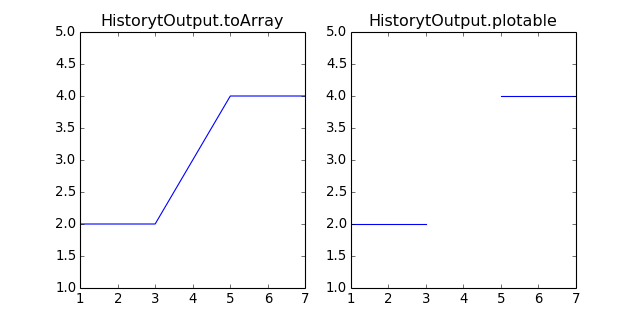`HistoryOutput.``toArray`()[source]

Returns an array.array of concatenated steps for x and y.

Return type: array.array
```>>> from abapy.postproc import HistoryOutput
>>> time = [ [1., 2.,3.] , [3.,4.,5.] , [5.,6.,7.] ]
>>> force = [ [2.,2.,2.] , [3.,3.,3.] , [4.,4.,4.] ]
>>> Force = HistoryOutput(time, force)
>>> x,y = Force.toArray()
>>> x
array('f', [1.0, 2.0, 3.0, 3.0, 4.0, 5.0, 5.0, 6.0, 7.0])
>>> y
array('f', [2.0, 2.0, 2.0, 3.0, 3.0, 3.0, 4.0, 4.0, 4.0])
```

#### Utilities¶

`HistoryOutput.``total`()[source]

Returns the total of all data.

Return type: float
`HistoryOutput.``integral`(method='trapz')[source]

Returns the integral of the history output using the trapezoid or Simpson rule.

Parameters: method (string) – choice between trapezoid rule (‘trapz’) or Simpson rule (‘simps’). float
```>>> from abapy.postproc import HistoryOutput
>>> time = [ [0., 1.], [3., 4.] ]
>>> data = [ [.5, 1.5], [.5, 1.5] ]
>>> hist = HistoryOutput(time = time, data = data)
>>> hist.integral()
1.0
>>> hist.integral()
1.0
>>> hist.integral()
2.0
>>> N = 10
>>> from math import sin, pi
>>> time = [pi / 2 * float(i)/N for i in xrange(N+1)]
>>> data = [sin(t) for t in time]
>>> hist = HistoryOutput()
>>> hist.add_step(time_step = time, data_step = data)
>>> trap = hist.integral()
>>> simp = hist.integral(method = 'simps')
>>> trap_error = (trap -1.)
>>> simp_error = (simp -1.)
```
Relative errors:
• Trapezoid rule: -0.21%
• Simpson rule: 0.00033%

Note

uses `scipy`

`HistoryOutput.``average`(method='trapz')[source]

Returns the average of all data over time using `integral`. This average is performed step by step to avoid errors due to disconnected steps.

Parameters: method (string) – choice between trapezoid rule (‘trapz’) or Simpson rule (‘simps’). float
```>>> from abapy.postproc import HistoryOutput
>>> from math import sin, pi
>>> N = 100
>>> hist = HistoryOutput()
>>> time = [pi / 2 * float(i)/N for i in xrange(N+1)]
>>> data = [sin(t) for t in time]
>>> hist.add_step(time_step = time, data_step = data)
>>> time2 = [10., 11.]
>>> data2 = [1., 1.]
>>> hist.add_step(time_step = time2, data_step = data2)
>>> sol = 2. / pi + 1.
>>> print 'Print computed value:', hist.average()
Print computed value: 1.63660673935
>>> print 'Analytic solution:', sol
Analytic solution: 1.63661977237
>>> print 'Relative error: {0:.4}%'.format( (hist.average() - sol)/sol * 100.)
Relative error: -0.0007963%
```
`HistoryOutput.``data_min`()[source]

Returns the minimum value of data.

Return type: float
`HistoryOutput.``data_max`()[source]

Returns the maximum value of data.

Return type: float
`HistoryOutput.``time_min`()[source]

Returns the minimum value of time.

Return type: float
`HistoryOutput.``time_max`()[source]

Returns the maximum value of time.

Return type: float
`HistoryOutput.``duration`()[source]

Returns the duration of the output by computing max(time) - min(time).

Return type: float

### `GetHistoryOutputByKey` function¶

`abapy.postproc.``GetHistoryOutputByKey`(odb, key)[source]

Retrieves an history output in an odb object using key (U2, EVOL, RF2,...)

Parameters: odb (odb object) – Abaqus output database object produced by odbAcces.openOdb. key (string) – name of the requested variable (i. e. ‘U2’, ‘COOR1’, ...) dict of HistoryOutput instance where keys are HistoryRegions names (i. e. locations)
```>>> from odbAccess import openOdb
>>> odb = openOdb('mySimulation.odb')
>>> from abapy.postproc import GetHistoryOutputByKey
>>> u_2 = GetHistoryOutputByKey(odb,'U2')
```

## Mesh¶

### `GetMesh` function¶

`abapy.postproc.``GetMesh`(odb, instance, dti='I')[source]

Retrieves mesh on an instance in an Abaqus Output Database.

Parameters: odb (odb object) – output database instance (string) – instance name declared in the Abaqus inp file. dti ('I' or 'H') – int data type in array.array Mesh instance
```from abapy.postproc import GetMesh
from odbAccess import openOdb
odb = openOdb('myOdb.odb')
mesh = GetMesh(odb,'MYINSTANCE')
```